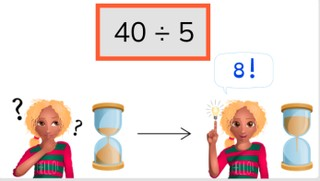Automaticity of division tables

# Automaticity of division tables

Students learn to memorize the division tables of 1 to 10.8,000 schools use Gynzy92,000 teachers use Gynzy1,600,000 students use Gynzy

## General

The students learn to memorize the division tables.

## Standards

CCSS.Math.Content.3.OA.C.7

## Learning objective

Students will be able to memorize the division tables of 1 to 10 and can quickly solve problems from them (automatically).

## Introduction

Have the students divide the money between the children. How much money does each child get?

## Instruction

Have the students solve the division problems within 5, 4, and 3 seconds. Next you show the pile of cards. Explain that you are going to start the timer and drag the cover away to reveal the first division problem. Have the students quickly give the correct answer to the problem and then drag away the card for the next problem. Try to do this within the time given.

Check whether the students have memorized the division tables with the following question:
- Why is it important to memorize the division tables?

## Quiz

The students test their memorization of the division tables with three exercises. In each exercise they must answer division flashcards within the given time.

## Closing

Discuss once again the importance of memorizing the division tables. Have the students make cards with problems from the division tables of 1 to 10. Have them write the problem on the front and the answer on the back. Now one of the student reads one of the cards out loud. The one that gives the correct answer first gets the card. Now another student reads a problem out loud and the other students can answer. The student with the most cards in the end wins the round.

## Teaching tips

Have student that have difficulty with memorizing the division tables first practice with solving the division tables of 1 to 10. When they are familiar with the individual division tables they can practice memorizing the division tables and answering problems from different division tables.

### The online teaching platform for interactive whiteboards and displays in schools

• Save time building lessons

• Manage the classroom more efficiently

• Increase student engagement